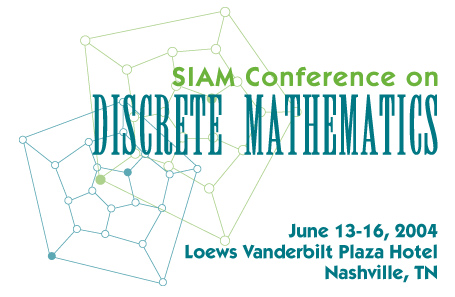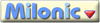Site best viewed with IE 6.0, Netscape 6.0, or Mozilla 1.4 or better

### What Makes a Finite Network High-Dimensional: Random Graph Scaling for Finite Graphs

Jennifer Chayes
Microsoft Corporation

Many models of practical interest, such as faulty wireless networks, are well described by random subgraphs of finite graphs, or more probabilistically, by percolation on finite graphs. For both theoretical and practical reasons, one of the key properties of these models is the behavior of the largest connected component as the underlying edge density is varied. While this behavior is well understood for the complete graph in the context of random graph theory, not much was known for general finite graphs.

In this work we formulate a sufficient condition under which transitive finite graphs exhibit the same scaling behavior as the complete graph, i.e., the same size of the dominant component and width of the critical window. Our condition is related to a well-known condition in percolation theory on infinite graphs, and more generally in field theories of mathematical physics, where it characterizes high-dimensionality. We verify this condition for many graphs of interest including the n-cube and the Hamming cube. Our techniques are a combination of methods from mathematical physics, in particular the lace expansion, and methods from combinatorics

This work is in collaboration with Christian Borgs, Gordon Slade, Joel Spencer and Remco van der Hofstad.

2/9/04
Dynamic menus by